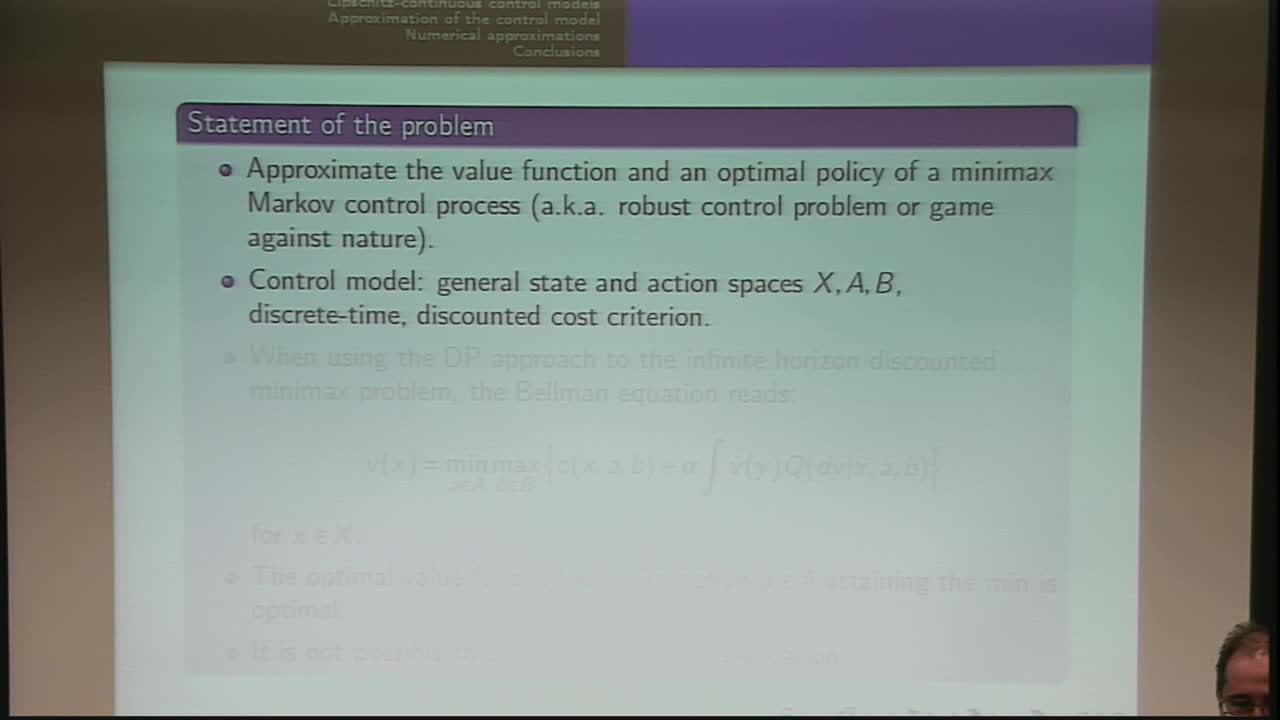## Numerical Approximations for Minimax Markov Control Problems

May 9, 2018
IMA
Presenters: Tomás Prieto Rumeau

Watch Video

#### Abstract

We study a minimax control problem with general state and action spaces under the discounted cost optimality criterion. We are interested in approximating numerically the value function and an optimal strategy of this general discounted minimax control problem. To do so, we provide an approximating minimax control model with finite state and action spaces, and hence computationally tractable. The discretization procedure of the state space is related to a probabilistic criterion based on the Wasserstein distance, while the action spaces are discretized with a geometric criterion, based on the Hausdorff metric. These results can be extended to two-player zero-sum Markov games.